# Python条件语句

...Python大约 3 分钟

1. `if` 结构
2. `if` - `else` 结构
3. `if` - `elif` 结构
4. `if` - `elif` - `else` 结构
5. 套娃式玩法

### # if 结构

if语句的格式是

``````if 条件:
如果条件成立执行的操作
``````

Python中并没有C语言那样的 `{ }`，而是用缩进来实现C语言中类似 `{ }` 的功能，一般按下 键盘上的`Tab`键即可完成缩进（或者四个空格）。缩进对于Python来说十分重要，如果使用不当，可能会引发错误。上面学习的 if语句 就使用到了缩进。

``````age = int(input("你的年龄是: "))
if age >= 18:
print("你已经成年")
``````

``````age = int(input("你的年龄是: "))
check = age >= 18
if check:
print("你已经成年")
``````

### # if - else 结构

``````if 条件:
如果条件成立执行的操作
else:
如果条件不成立执行的操作
``````

``````if not 条件:
如果条件不成立执行的操作
else:
如果条件成立执行的操作
``````

``````age = int(input("你的年龄是: "))
if age >= 18:
print("你已经成年")
else:
print("未成年")
``````

### # if - elif 结构

``````if 条件1:
如果条件1成立执行的操作
elif 条件2:
如果条件1不成立但是条件2成立执行的操作
``````

``````age = int(input("你的年龄是: "))
if age >= 18:
print("你已经成年")
elif age <18:
print("未成年")
``````

### # if - elif - else 结构

``````if 条件1:
如果条件1成立执行的操作
elif 条件2:
如果条件1不成立但是条件2成立执行的操作
else:
如果条件不成立执行的操作
``````

Python执行命令的顺序一般情况下是自上而下，所以条件1和条件2都满足的情况下会优先执行条件1，然后结束条件语句的线程。

``````age = int(input("你的年龄是: "))
if age in range(0,18):  # range区间的范围为左闭右开
print("未成年")
elif age >= 18:
print("成年")
else:
print("数值有误")
``````

`range` 区间的范围为左闭右开，如`range(0，19)`表示数学中的`[0,19)`

### # 套娃式玩法

``````age = int(input("你的年龄是: "))
if age < 0:
print("数值有误")
else:
if age < 18:
print("未成年")
elif age >= 18:
print("成年")
``````

•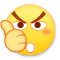0
•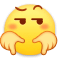0
•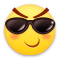0
•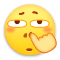0
•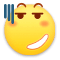0
•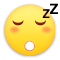0

• 按正序
• 按倒序
• 按热度